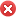#### 湖南城市学院湖南益阳市

• 官方网址：http://www.hncu.net/
• 官方电话：0737-4628168,0737-6355818
• 电子邮箱：zsc@hnist.edu.cn

## 招生快讯

#### 湖南城市学院2020年招生章程

\r

\r

\r

\r

\r

\r

\r

\r

\r

\r

\r

\r

\r

\r

\r

\r

\r

\r

\r

\r

\r

\r

\r

\r

\r

\r

\r

\r

\r

\r

\r

\r

\r

\r

\r

\r

\r

\r

\r

\r

\r

\r

\r

\r

\r

\r

\r

\r

\r

\r

\r

\r

\r

\r

\r

\r

\r

0737-6110025（纪委办/省监委驻校监察专员办）

\r

\r

## 男女比例63%37%98.6%

16.5%

4.8%

## 同类院校推荐## 报考指南